This blog is managed by Song Hock Chye, author of Improve Your Thinking Skills in Maths (P1-P3 series), which is published and distributed by EPH.

## Wednesday, December 23, 2009

### Improve Your Thinking Skills in MathsThe first set in the series, "Improve Your Thinking Skills in Maths" (Primary 3) which I wrote, has been released by Singapore's biggest publisher of school textbooks, Educational Publishing House (EPH). The other 2 series, which I also wrote (Primary 1 and 2), will be out soon.

The above series can be purchased at Popular outlets.

Regards

## Sunday, December 20, 2009

Our son completed his OCS on 19 Dec 09 and was commissioned an officer in the SAF.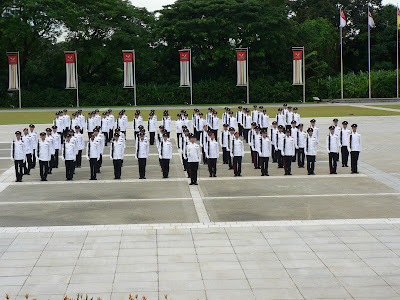Related News
More than 500 SAF cadets commissioned as officers

SINGAPORE: More than 500 officer cadets were commissioned as officers at the SAFTI Military Institute on Saturday evening.

Deputy Prime Minister and Minister for Defence Teo Chee Hean reviewed the commissioning parade for 402 Army, 52 Navy and 50 Air Force officer cadets.

The parade marked the completion of 38 weeks of rigorous training, during which the officer cadets were put through realistic training scenarios to develop their combat, planning and leadership skills.

The newly commissioned SAF officers will assume operational, instructional or staff appointments.

Addressing the graduands at the parade, Mr Teo encouraged them to take responsibility of the training, morale and discipline of their men to ensure that they were operationally ready at all times.

"As their commander, make good soldiers out of them... win over the hearts and minds of your men, for it is the resilience and fighting spirit of our people that will ultimately make the difference," said Mr Teo.

Education Minister and Second Minister for Defence Ng Eng Hen, Members of Parliament, senior Ministry of Defence officials and SAF officers, as well as families and friends of the newly commissioned officers also attended the parade.

- CNA/so

## Friday, November 27, 2009

### Nobody Video Clip

Now that PSLE is over, it's time for some light entertainment.

A funny video clip about mom's advice that you should always check what you need before doing things. That aside, it is a popular video clip judging by the ratings it scored and the number of views it recorded.

Nobody But You Full Music Video - Wonder Girls (HQ)

## Monday, November 23, 2009

### PSLE results to be released on Thursday

From Channel News Asia

PSLE results to be released on Thursday
Posted: 23 November 2009 1215 hrs

SINGAPORE: The Primary School Leaving Examination (PSLE) results will be out on Thursday.

Pupils can get their result slips from their schools from noon on November 26. Eligible pupils will also receive their option forms to select their secondary schools.

With the PIN contained in the sealed option form, parents can submit their child's secondary school options online via the Secondary One Internet System.

The system is accessible 24 hours a day through the MOE Secondary One Posting website, starting from noon on Thursday. Application closes at 3pm on December 2.

Alternatively, parents may also submit their child's secondary school options through their child's primary school.

The Secondary One posting results will be released on Tuesday, December 22. Pupils are to report to their secondary schools on Wednesday, December 23, at 8.30am.

- CNA/so

## Monday, October 26, 2009

### Tuition Classes for Academic Year 2010

Please note that we will start our new tuition classes for the Academic Year 2010 on 14 Nov 2009.

Our contact details can be found in this link.

## Sunday, October 11, 2009

### Simple Machines - The Movable Pulley

One of the functions of simple machines is to lighten our workload. A small effort can move a greater load. What is sacrificed is that the distance moved by the effort is greater than the distance moved by the load.

As an example, we will take a look at the movable pulley. So how does a movable pulley help to lighten our work?

Remember that in order to be able to move a load that is greater than the effort, the distance moved by the effort has to be greater than the distance moved by the load.With reference to the diagram above:-

Pulley A - The distance from the pulley to the ceiling is 2m. This means that the cable attached to the pulley is also 2m.

Pulley B – The distance moved by the effort is 2 m. However, the load moves only 1 m from the original position of the pulley. This is confirmed by …. 2-m cable divided by 2 = 1m, which means that the distance from the pulley to the ceiling is 1m. Hence, the distance from the pulley now, is 1 m from where it was originally.

Since the effort moved twice the distance moved by the load, only ½ the effort is needed to raise the load. Hence if the load weighs 10 kg, an effort of slightly over 5 kg only is needed to move the load.

### Simple Machines – The Inclined Plane

As with all machines, if we want the force exerted by the effort to be less than the force exerted by the load, the distance travelled by the effort has to be more than the distance travelled by the load.

Below are 4 illustrations how the inclined plane works.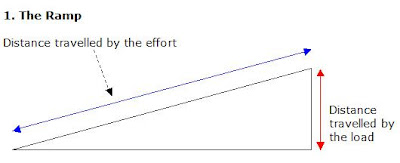The more gradual the slope, the greater the distance travelled by the effort compared to the distance travelled by the load. This means that the more gradual the slope, the less effort is needed to move the load.

Examples of applications of the ramp in real life – wheelchair ramps for the disabled, gradual and winding slopes of a road found in mountainous terrain (e.g. roads to Gentling Highlands or Cameron Highlands).It can be noted that the ‘sharper’ the wedge, the less the effort is needed because for the same distance travelled by the effort, the distance moved by the load is now less.

Examples of applications of the wedge in real life – axe head, blades of knives, metal wedges for prying open flanges of pipes in heavy industries.Fig 1 - For every 1 turn the screw makes, the screw is driven down by the distance of ‘1 pitch’. Hence, the more gradual the slope of the thread, the smaller the distance it will be between 2 threads, as shown in Fig 2.

Fig 2 - the distance travelled by the load is less than in Fig 1, although in both cases, the effort moves by the same one turn. Since the load moves less in Fig 2, less effort is also needed.

In conclusion, for screws, the more the number of threads there is, OR the smaller the pitch, OR the more gradual the slope of the threads, the less the effort is needed to move the load.Again, like the screw, the smaller the pitch, the less the ends of the ‘V’ move towards each other, which also means the less the distance the load moves.

Like the screw, for the screw jack, the more the number of threads there is, OR the smaller the pitch, OR the more gradual the slope of the threads, the less effort is needed to move the load.

Take note that for the screw jack, while the mechanism of the inclined plane is used to lift the vehicle off the ground, the handle of the screw jack works on the principle of the wheel and axle. In this case, the handle is the wheel, while the screw of the jack (the part with the threads) is the axle.

Important note to parents and students – The purpose of the above 4 illustrations is to help students understand the mechanics of the inclined plane. Most schools’ practice papers and science textbooks do not use the terms ‘pitch’ or ‘threads’. Those terms are technical terms used in the heavy and light industries.

In trying to make the illustration as simple as possible, I have found that I cannot avoid using those technical terms. The use of the above terms is to assist the student (or parent) to understand the mechanics of screws and screw jacks.

Take note that markers who will be marking your Science PSLE Paper, in all likelihood have been teachers all their lives and may not have worked in industries before, and would probably not be familiar with the terms ‘pitch’ and ‘threads’.

In other words, it is highly advised that you do not use the terms ‘pitch’ and ‘threads’, unless you are able to draw and label the diagrams as accurately as the above.

## Saturday, October 10, 2009

### Parents up in arms again over PSLE Mathematics paper

From Channel News Asia

As requested by some students, here is one possible workout for the solution.

Question

Jim bought some chocolates and gave half of it to Ken. Ken bought some sweets and gave half of it to Jim. Jim ate 12 sweets and Ken ate 18 chocolates. The ratio of Jim's sweets to chocolates became 1:7 and the ratio of Ken's sweets to chocolates became 1:4. How many sweets did Ken buy?

Solution

Chocolates ----- C
Sweets ----- S

Jim bought 2 units of chocolates ----- C, C
Ken bought 2 units of sweets ---- S, S

Jim gave 1 unit of chocolates to Ken and Ken gave 1 unit of sweets to Jim.
Jim ----- S, C
Ken ----- S, C

Jim ate 12 sweets and Ken ate 18 chocolates.
Jim ----- S – 12; C
Ken ----- S; C – 18

Jim’s sweet to chocolate ratio 1:7. Therefore for number of sweets to be same as number of chocolates, multiply sweets by 7.
C ----- 7 x (S – 12)
C ----- 7S – 84

Ken’s sweet to chocolate ratio 1:4. Therefore, for number of sweets to be same as number of chocolates, multiply sweets by 4.
4 x S ---- C – 18
4S ---- C – 18

From Jim, (C ----- 7S – 84), replace Ken's C....
(Ken) 4S ----- C - 18
4S ----- (7S – 84) – 18
4S ----- 7S – 102
3S ----- 102
1S ----- 34

Number of sweets Jim had at first
2 units of sweets ----- 2 x 34 = 68

## Monday, September 28, 2009

### Rosyth Sch 2007 PSLE Math Prelim Q48

A bus was travelling at a constant speed from Town A to Town B. It passed a car travelling at a constant speed of 90 km/h in the opposite direction. 1 and 1/2 hours later, the bus reached Town B but the car was still 25 km away from Town A. If the bus took 4 hours to complete the whole journey, what is the distance between the two towns?

Solution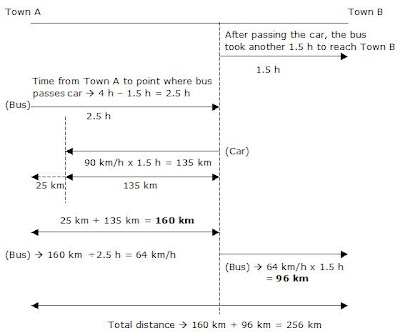### Rosyth Sch 2007 PSLE Math Prelim Q47

The perimeter of a rectangle to that of a square is in the ratio of 11:6. If the square has an area of 144 square m and the length and breadth of the rectangle are in the ratio of 6:5, find the length and breadth of the rectangle.

Solution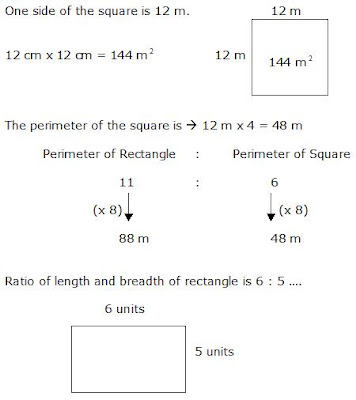Perimeter
(6 + 6 + 5 + 5) units ----- 88 m
22 units ----- 88 m
1 unit ----- 88m divided by 22 = 4 m

(Length) 6 units ----- 6 x 4 m = 24 m
(Breadth) 5 units ----- 5 x 4 m = 20 m

### Rosyth Sch 2007 PSLE Math Prelim Q46

The total cost of 28 textbooks and workbooks is \$784.
3/4 of the books are textbooks and the remaining books are workbooks. A workbook cost half as much as a textbook. Find the difference in the price of a textbook and a workbook.

Solution

Workbook ----- 1 unit
Textbook ------ 2 units

3/4 of the books are textbooks ----- 3/4 x 28 = 21

Workbooks ----- 28 – 21 = 7

21 textbooks + 7 workbooks ---- \$784

(21 x 2 units) + 7 units ----- \$784

42 units + 7 units ----- \$784

49 units ----- \$784

1 unit ------ \$784 divided 49 = \$16

## Friday, September 25, 2009

### Rosyth Sch 2007 PSLE Math Prelim Q45

The figure shows a cube with 3 painted parts A, B and C. These painted parts are of the same area and they are touching the midpoints of the sides of the cube. The total area of the painted parts is 54 square cm. Find the volume of the cube.3 painted parts on 3 faces of the cube ----- 54 square cm

1 painted part on 1 face of the cube
54 square cm divided by 3 = 18 square cm

Area of 1 triangle is 18 square cm divided by 2
= 9 square cm

For area of triangle to be 9 square cm, the base has to be 6 cm and height has to be 3 cm as worked out below
(1/2 x 6 cm x 3 cm = 9 square cm)

1 side of the cube is therefore 6 cm as seen from above diagram.

Volume of cube
(6 x 6 x 6) cubic cm = 216 cubic cm

### Rosyth Sch 2007 PSLE Math Prelim Q44

The figure is not drawn to scale. What fraction of the figure is the total area of the shaded regions A, B, C, D, E, F, G and H?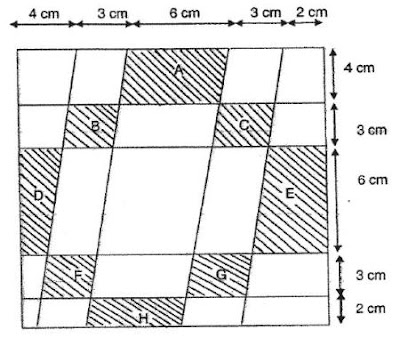Solution

The base of the whole figure is (4 + 3 + 6 + 3 + 2) cm = 18 cm

1st row from the top
Fig A has a base of 6 cm.

2nd row
The sum of the bases of Figs B and C is 6 cm.

3rd row
The sum of the bases of Figs D and E is 6 cm.

4th row
The sum of the bases of Figs F and G is 6 cm.

5th row
Fig H has a base of 6 cm.

The average length of the bases of all shaded figures is 6 cm.

The length of the base of the whole figure is 18 cm.

Therefore, the fraction of whole figure that is shaded is
6 cm divided by 18 cm = 1/3

Note that the above method can be used because the vertical columns also correspond to the 6 cm shaded out of the 18 cm total vertical length of the figure.

### Rosyth Sch 2007 PSLE Math Prelim Q43

The area of the figure ABCD is 48.5 square cm . Find the length of BE.Solution

Area of ACD
1/2 x 18 cm x 10.4 cm = 93.6 square cm

Area of ABC
93.6 square cm - 48.5 square cm = 45.1 square cm

Area of Triangle ABC ----- 1/2 x base x height
45.1 square cm = 1/2 x 18 cm x height

height = (45.1 sq cm x 2 ) divided by18 cm
= 5.01 cm ~ 5.0 cm (to the nearest tenth)

### Rosyth Sch 2007 PSLE Math Prelim Q41

The table below shows the rates at which a construction worker is being paid daily.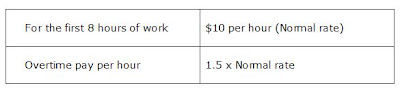How many hours must he work in a day to earn \$140?

Solution

First 8 hours of work ----- \$10 x 8 = \$80

To earn \$140, he needs another \$60.

Overtime rate is ----- \$10 per hour x 1.5 = \$15 per hour.

For overtime pay to be equal to \$60, he must work
\$60 divided by \$15 per hour = 4 hours

Total time he needs to work
8h + 4h = 12h

## Thursday, September 24, 2009

### Rosyth Sch 2007 PSLE Math Prelim Q40

Machine A can do a printing job in 2 hours. Machine B can do the same job in 3 hours. If both machines are used at the same time, how long would it take to complete half the printing job?

Solution

Since Machine A takes 2 hours and Machine B takes 3 hours, Machine A will be able to get more work done than Machine B, if both are used at the same time, in the ratio of 3:2 as shown below.(Machine A)
Whole job ----- 2 hours
3/5 of job ----- 3/5 x 2 hours = 6/5 hours

Time taken if both machines are used at the same time is 6/5 hours because while Machine A completes 3/5 of the job, Machine B completes the remaining 2/5 of the job.

Time taken for whole job done if both machines are used at same time is therefore 6/5 hours

Time taken for half the job done if both machines are used at the same time is
1/2 x 6/5 hours = 3/5 hour.

### Rosyth Sch 2007 PSLE Math Prelim Q39

Find the sum of the six marked angles in the diagram.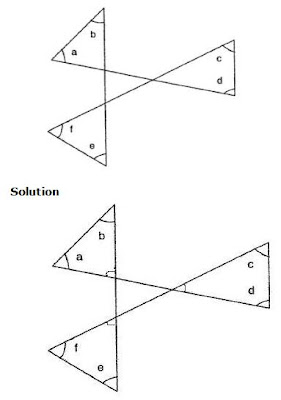The sum of the all the angles in the triangle in the centre is 180 degrees. The sum of all the 3 angles outside the centre triangle, in the figure above is therefore also 180 degrees (opposite angles).

The sum of all the six marked angles is hence,

Sum of marked angles in 1 triangle ----- 180 degrees
Sum of marked angles in 3 triangles ----- 180 degrees x 3 = 540 degrees
Sum of 6 marked angles ----- (540 – 180) degrees = 360 degrees

## Wednesday, September 23, 2009

### Rosyth Sch 2007 PSLE Math Prelim Q38

In the figure not drawn to scale, the ratio of the area of the bigger square to the smaller square is 8:4. If 25% of the larger square is shaded, what percentage of the whole figure is not shaded?Solution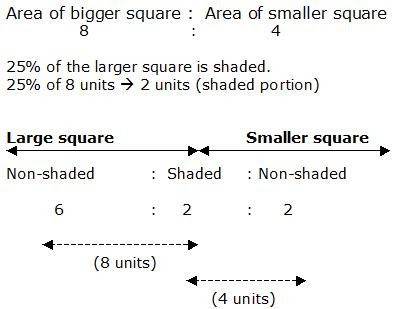Portion unshaded ----- 6 units + 2 units = 8 units
Total area ----- 6 units + 2 units + 2 units = 10 units

Percentage of figure that is not shaded
8/10 x 100% = 80%

### Rosyth Sch 2007 PSLE Math Prelim Q37

A tank with a square base of side 5 m was 1/3 full of water. When another 100 cubic meters of water was poured into the tank, it became 1/2 full. Find the capacity of the tank.

Solution

1/2 capacity – 1/3 capacity = 1/6 capacity

1/6 capacity ----- 100 cubic metres

6/6 capacity ----- 6 x 100 cubic m = 600 cubic m

### Rosyth Sch 2007 PSLE Math Prelim Q36

The average weight of 5 boys is 8w kg. When 2 more children whose weights are 11w kg and 12 w kg respectively joined the group, what is the average weight of the boys now?

Solution

Average weight of 5 boys ----- 8w kg

Total weight of 5 boys ----- 8w kg x 5 = 40w kg

Total weight of 7 boys
(40w + 11w + 12w) kg = 63w kg

Average weight of 7 boys
63w kg divided by 7 = 9w kg

## Tuesday, September 22, 2009

Excel Eduservice would like to thank everyone for supporting this website. We are pleased this site has helped many P6 students, parents and tutors the last 2 years.

Free Maths Worked Solutions and PLSE Aggregate Score Calculator

There is a NEW free maths worked solution (Nanyang Pri P6 Prelim 2007) in the free section above.

At Excel Eduservice, we work to serve you better.

You're in good hands when you're with Excel!

### Raffles Girls Pri Sch 2007 PSLE Math Prelim Q48

Valley Department Store sold a dress for \$585. This was 17% more than the price of a similar dress in Wiki Department Store. During a sale, both stores offered same percentage discount on the dress. Christine bought the dress in Wiki Department Store and found that she paid \$68 less than the discounted price in Valley Department Store.

(a) Find the price of the dress in Wiki Department Store before the sale.
(b) What is the percentage discount given during the sale?

Solution

(a)

Valley ----- 100% + 17% = \$585
117% ----- \$585
1% ----- \$585 divided by 117 = \$5

(Wiki) 100% ----- 100 x \$5 = \$500

(b)
Before discount\$85 is the difference in price between Valley and Wiki before the discount. After the discount, the difference was \$68.

\$85 - \$68 = \$17
Discount ----- (\$17/\$85) x 100% = 20%

### Raffles Girls Pri Sch 2007 PSLE Math Prelim Q47

Melissa spent 3/8 of her money on 3 pencils and 8 pens, and 4/5 of the remainder on 15 markers. Each pencil cost 2/3 as much as a pen. Each marker cost \$0.20 more than a pencil. What is the cost of a pen?

Solution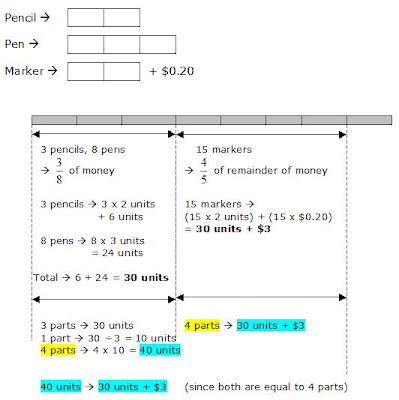40 units – 30 units ----- \$3
10 units ----- \$3
1 unit ----- \$3 divided by 10 = \$0.30

(Pen) 3 units ----- \$0.30 x 3 = \$0.90

## Monday, September 21, 2009

### Raffles Girls Pri Sch 2007 PSLE Math Prelim Q46

At first, the ratio of the number of marbles received by John and Peter was 4:7. The ratio of the number of marbles received by Peter and Sam was 9:5. Then, John gave 1/12 of his marbles to Sam, and Peter gave 1/9 of his marbles to Sam. As a result, Sam had 135 marbles in the end.

(a) Find the ratio of the number of John’s marbles to the number of Sam’s marbles at first.

(b) Find the total number of marbles received by the 3 boys.

Solution45 units ----- 135 (marbles)
1 unit ----- 135 divided by 45 = 3
134 units ----- 134 x 3 = 402

## Thursday, September 17, 2009

### Raffles Girls Pri Sch 2007 PSLE Math Prelim Q45

The figure below shows a rectangle PQRS. The lines are extended from point P, Q, R and S and they meet at point Y. The length of QR is 20 cm and the length of XY is 4 cm. Given that QS is a straight line, the area of Triangle PQY is 72 square cm and the area of Triangle SRY is 84 square cm, find the shaded area of Triangle QSY.Solution

Area of Triangle PQY + Area of Triangle SRY
72 sq cm + 84 sq cm = 156 square cm

Area covered by Triangles PQY and SRY is also 1/2 of area of Rectangle PQRS. This also means that Area of Triangle PQS is also 156 sq cm.

Area of Triangle PSY = 1/2 x 20 cm x 4 cm = 40 sq cm

Area of Triangle PQY = 72 sq cm

Area of PQS – Area of PQY – Area of PSY
=156 sq cm - 72 sq cm - 40 sq cm= 44 square cm

### Raffles Girls Pri Sch 2007 PSLE Math Prelim Q44

A group of pupils sat for two tests, test A and test B. The number of pupils who failed test A was 1/7 of those who passed test A. Given that there were 20 pupils who failed test A,

(a) How may pupils passed test A?
(b) The number of pupils who failed test B was 1/4 of those who failed test A. What fraction of the pupils passed test B?

Solution(a)
1 unit ----- 20
7 units ----- 20 x 7 = 140

(b)
Failed Test A ----- 20
Failed Test B ----- 1/4 x 20 = 5

(Total number of pupils)
8 units ----- 8 x 20 = 160

Fraction of pupils who failed Test B
5/160 = 1/32

Fraction of pupils who passed Test B
1 – 1/32 = 31/32

## Wednesday, September 16, 2009

### Raffles Girls Pri Sch 2007 PSLE Math Prelim Q43

There are some red and green beads in a container. If 75 more green beads are put into the container, the percentage of red beads will decrease from 30% to 20%. How many red beads are in the container?

Solution12 units ----- 7 units + 75
5 units ----- 75
1 unit ----- 75 divided by 5 = 15

Red at first
3 units ----- 3 x 15 = 45

## Tuesday, September 15, 2009

### Raffles Girls Pri Sch 2007 PSLE Math Prelim Q41

Jane started driving at 9.30 am from Town A to Town B. At 11.30 am, Jane had covered only 2/5 of the distance. She had to cover another 144 km before she reached Town B.

(a) What was the distance between Town A and Town B?

(b) If Jane were to travel at an average speed of 72km/h after 11.30 am, at what time would she reach Town B?

Solution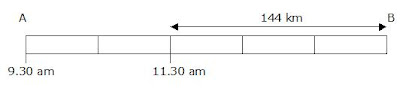(a)
3 units ----- 144 km
1 unit ----- 144 km divided by 3 = 48 km
5 units ----- 5 x 48 km = 240 km

(b)
Time = 144km divided by 72 km/h = 2 h
2 hours after 11.30 am is 1.30 pm or 1330 h.

## Monday, September 14, 2009

### Raffles Girls Pri Sch 2007 PSLE Math Prelim Q40

A rectangular tank with 5 identical solid metal cubes was filled to the brim with water. Water was drained out of the tank through a tap at a rate of 2 litres per minute. It took 18 minutes for the water level to drop to the height of the metal cubes. Find the volume of the 5 metal cubes.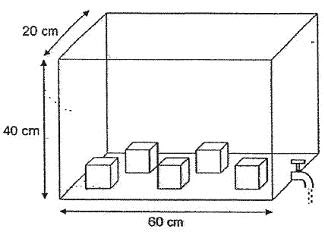Solution

Volume of whole tank
60 cm x 20 cm x 40 cm = 48 000 cubic cm or 48 litres

1 min ----- 2 litres

18 min ----- 2 litres x 18 = 36 litres

Volume of tank filled with water + volume of cubes after 18 min
48 litres – 36 litres = 12 litres or 12 000 cm

Height of cubes is therefore,
Height ------ Volume divided by base area
= 12 000 cubic cm divided by (60 cm x 20 cm) = 10 cm

Height of cube ------ 10 cm
Volume of 1 cube ----- 10 cm x 10 cm x 10 cm = 1000 cubic cm
Volume of 5 cubes ----- 1000 cubic cm x 5 = 5000 cubic cm

## Wednesday, September 09, 2009

### Anglo Chinese School 2007 PSLE Math Prelim Q48

Mr Goh was travelling from Town X to Town Y. After completing 2/7 of the journey, he passed by Mr Lee travelling the same direction. Mr Lee was travelling at an average speed of 60 km/h. Mr Goh reached his destination 3 hours later, while Mr Lee was still 45 km away from Town Y.

(a)Find the distance between the two towns.
(b)If Mr Lee left Town x at 11.30 am, what time would he arrive at Town Y?

Solution(a)
(Goh) 5/7 of journey ----- 225 km
1/7 of journey ----- 225 km 5 = 45 km
(Whole journey) 7/7 ----- 45 km x 7 = 315 km

(b)
Lee’s time ----- distance divided by speed
315 km divided by 60 km/h
= 5.25 hours or 5 hours 15 min

Since Lee left at 11.30 am, he would arrive at 4.45 pm.

## Tuesday, September 08, 2009

### Anglo Chinese School 2007 PSLE Math Prelim Q47

In an auditorium, the ratio of the number of competitors to the number of non-competitors is 8:5. The ratio of the number of male competitors to the number of female competitors is 7:4. Given that 3/5 of the non-competitors are males and there are 32 female competitors, how many males and females are there in the auditorium?

SolutionMales ----- 56 + 33 = 89
Females ----- 32 + 22 = 54

### Anglo Chinese School 2007 PSLE Math Prelim Q46

A rectangular container measuring 45 cm by 10 cm by 20 cm is empty at first. Water flows from Tap X at a rate of 1.3 litres per minute and from Tap Y at a rate of 1.2 litres per minute. How long does it take to completely fill up the container if both taps are turned on at the same time? Give your answer in minutes and seconds.

Solution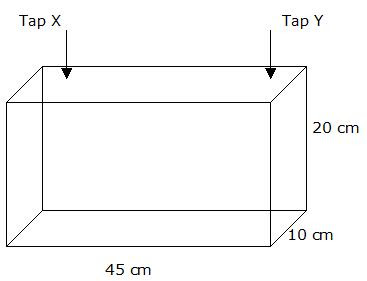Volume of tank ----- 45 cm x 10 cm x 20 cm
= 9000 square cm or 9 litres

1 min ----- 1.3 litres/min + 1.2 litres/min = 2.5 litres/min

9 litres divided 2.5 litres/min = 3.6 min or 3 min 36 seconds

## Monday, September 07, 2009

### Anglo Chinese School 2007 PSLE Math Prelim Q45

The figure is made up of three circles and two semi-circles. O is the center of the circle. AB is 42 cm. Find the total area of the shaded part.Area of 1 square ----- (10.5 x 10.5)square cm = 110.25 square cm

Area of 2 squares ----- 2 x 110.5 square cm = 220.5 square cm

Area of shaded area is ---- Area of whole figure – area of 1 small circle – area of 4 small quadrants – area of 2 squares

(1386 - 346.5 - 346.5 - 220.5) square cm = 472.5 square cm

## Sunday, September 06, 2009

### Anglo Chinese School 2007 PSLE Math Prelim Q44

Marvin bought a box of fruits. 30% of the fruits are apples and the rest are oranges. He realized that half of the apples were rotten and threw them away. He then bought some oranges and the number of oranges increased by 40%. After that, he found that there were 52 more fruits in the box. How many fruits were there in the box at first?

Solution

At first
Apples ----- 30%
Oranges ----- 70%

Threw half apples away, bought 40% more oranges,
Apples ----- 1/2 x 30% = 15%
Oranges ----- 70% + (40/100 x 70%) = 98%

Total ----- 15% + 98% = 113%

113% - 100% = 13% (increase in number of fruits)
13% ----- 52 fruits
1 % ----- 52% divided by 13 = 4

Number of fruits at first,
100% ----- 4 x 100 = 400

### Anglo Chinese School 2007 PSLE Math Prelim Q43

Derrick had 2/3 as many stickers as Benedict. After Derrick bought another 8 stickers and Benedict lost 5 stickers, Derrick now has 4/5 as many stickers as Benedict. Find the number of stickers Derrick and Benedict had at first.

Solution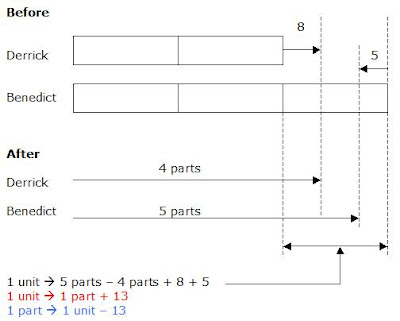(Derrick after)
4 parts ----- 2 units + 8
2 parts ----- 1 unit + 4
2 parts ----- 1 part + 13 + 4
2 parts – 1 part ----- 17
1 part ----- 17

1 unit – 13 ----- 17
1 unit ----- 17 + 13 = 30
5 units ----- 30 x 5 = 150

### Anglo Chinese School 2007 PSLE Math Prelim Q41

Alex has \$1.50 more money than Betty and three times as much money as Colin. The 3 of them have \$9.70 altogether. How much does Colin have?

Solution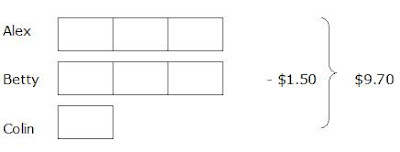7 units - \$1.50 ----- \$9.70

7 units ----- \$9.70 + \$1.50 = \$11.20

1 unit ----- \$11.20 divided by 7 = \$1.60

### Anglo Chinese School 2007 PSLE Math Prelim Q40

Fara started collecting stamps in January. In each month from February to May, she collected 30 stamps more than the month before. She saved a total of 750 stamps from January to May. How many stamps did she collect in January?

Solution5 units + 10 groups of 30 ---- 750

5 units + 300 ---- 750

5 units ---- 750 – 300 = 450

1 unit ---- 450 divided by 5 = 90

## Saturday, September 05, 2009

### Anglo Chinese School 2007 PSLE Math Prelim Q38

In the figure not drawn to scale, ABCD is a square. BDE and BCF are equilateral triangles. What is Angle FGE?Solution

Angle BCF ---- 60º (Triangle BCF is an equilateral triangle)

Angle CBD ---- 45º

Angle CBG ---- 60º - 45º = 15º

Angle BGC ---- 180º - 60º - 15º = 105º (sum of angles in a triangle)

Angle FGE ---- 105º (opposite angles)

## Wednesday, September 02, 2009

### Singapore Chinese Girls Sch 2008 PSLE Math Prelim Q48

At a farm, 40% of the animals are cows, 90% of the remainder are sheep and the rest are ducks. There are 56 more sheep than cows. After some cows died, 20% of the remaining animals at the farm are cows. How many cows are there left at the farm?

Solution

Cows ---- 40%
Remainder (sheep + ducks) ---- 100% - 40% = 60%

Sheep ---- 90% (remainder) x 60% = 54%

Sheep more than cows ---- 56
(Sheep) – (Cows) ---- 56
54% - 40% ---- 56
14% ---- 56
1% ---- 56 divided by 14 = 4

(Total) 100% ---- 100 x 4 = 400
(Sheep + ducks) ---- 60%
60% ---- 60 x 4 = 240

After some cows died 80% were sheep and ducks
80% ---- 240
1% ---- 240 divided by 80 = 3

(Cows left) 100% - 80% = 20%
20% ---- 20 x 3 = 60

Answer: There were 60 cows left.

## Sunday, August 30, 2009

### Singapore Chinese Girls Sch 2008 PSLE Math Prelim Q47

Mary had some \$5 and \$10 notes in her wallet. There was a total of 60 pieces of notes at first. She used half of the number of \$5 notes and received another twelve \$10 notes from her mother. After that, the number of \$10 notes she had was 2/3 of the remaining number of \$5 notes she had left. How much money did she have at first?

Solution

After she used half of her \$5 notes and mother gave her twelve \$10 notes:8 units - 12 ---- 60
8 units ---- 60 – 12 = 72
1 unit ---- 72 divided by 8 = 9

(\$5 notes) 6 units ---- 6 x 9 = 54
(\$10 notes) 2 units – 12 = (2 x 9) – 12 = 6

(54 x \$5) + (6 x \$10)
= \$270 + \$60

## Wednesday, August 26, 2009

### Singapore Chinese Girls Sch 2008 PSLE Math Prelim Q44

Andrew left Town X for Town Y which was 500 km apart. He travelled at an average speed of 90 km/h for 3/5 of the journey. He then increased his speed by 30 km/h for the rest of the journey and reached Town Y at 2 pm. Richard also left Town X for Town Y at the same time as Andrew and he drove at an average speed of 100 km/h for the whole journey.

a) What time did Andrew leave Town X?
b) How far apart were they at 1 pm?

Solution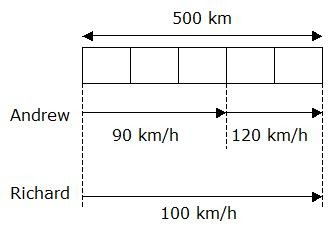5 units ---- 500 km
1 unit ---- 500 km divided by 5 = 100 km
Every 1/5 of journey ----- 100 km

(a)
Andrew’s Time (1st 3/5 of journey) ---- distance divided by speed
= 300 km divided by 90 km/h
= 3 and 1/3 hours

Andrew’s Time (last 2/5 of journey)
= 200 km/h divided by 100 km/h
= 1 and 2/3 hours

Total time taken by Andrew ----
(3 and 1/3 hours) + (1 and 2/3 hours) = 5 hours

He reached Town Y at 2 pm.
5 hours before 2 pm is 9 am.

Answer: Andrew left Town X at 9 am.

(b)
At 1 pm, both of them travelled for 4 hours.

Richard’s distance ----- speed x time
= 100 km/h x 4 h
= 400 km

Andrew’s distance at 1 pm was ----

For the 1st 3 and 1/3 hours, he travelled 3/5 of journey ---- 300 km.

For the next 2/3 hour he travelled ---- speed x time
= 120 km/h x 2/3 h
= 80 km

Andrew’s total distance in 4 hours ----
300 km + 80 km
= 380 km

They were apart by ----
400 km (Richard’s distance) – 380 km (Andrew’s distance)
= 20 km

Answer: They were 20 km apart.

### Singapore Chinese Girls Sch 2008 PSLE Math Prelim Q43

There were 9 chairs in each row. 8 rows of chairs were rearranged equally spaced, to form the perimeter of a square. There were same number of chairs on each side of the square. How many chairs were there on each side of the square?

Solution

Total number of chairs ---- 8 x 9 = 72
No. of chairs less 4 corners ---- 72 - 4 = 48
No. of chairs per row less 4 corners ---- 68 divided by 4 = 17
No. of chairs on 1 side ---- 17 + (2 corner chairs) = 19

## Friday, July 31, 2009

### Singapore Chinese Girls Sch 2008 PSLE Math Prelim Q42

In the diagram below, 75% of square Z is not shaded. The ratio of the area of square X to the area of square Y is 9:4. The ratio of the area of square Y to the area of square Z is 4:1.
a) What is the ratio of the area of square X to the area of square Z?
b) Find the ratio of the unshaded area of square Y to the unshaded area of square Z.Solution

a)
X : Y : Z
9 : 4 : 1

X : Z

b)
Total Area
Y : Z
4 : 1
16 : 4

Y : Z
15 : 3 * (see explanatory notes below)

* Total Area (ratio) Y:Z is 4:1. 75% of Z is unshaded, hence for Z, 3 units out of 4. This means 1 unit is shaded. For Y, 16 units total less 1 unit shaded = 15 units unshaded. Hence, unshaded area is Y:Z ---- 15:3.

## Thursday, July 30, 2009

### Singapore Chinese Girls Sch 2008 PSLE Math Prelim Q40

The number of girls who registered for art class was 20% of the number of boys. On the actual day, 3 more girls and 3 more boys turned up for the class. As a result, there were 1/3 as many girls as boys at the art class. What was the total number of children who came to art class?

Solution

Girls 20% of boys, hence, girls 1/5 of boys

Before

Girls 1 unit
Boys 5 units

After 3 more boys and 3 more girls turned up, there were 1/3 as many girls as boys:

Girls 1 unit + 3 ----- 1 part (x3)
Boys 5 units + 3 ----- 3 parts

Girls 3 units + 9 ----- 3 parts
Boys 5 units + 3 ----- 3 parts

(Boys) 5 units + 3 ----- 3 units + 9 (Girls)
5 units – 3 units ----- 9 – 3
2 units ----- 6
1 unit ----- 3

Total number of students

Girls ---- 3 + 3 = 6
Boys ---- (5 x 3) + 3 = 18

6 + 18 = 24

## Thursday, July 23, 2009

### Rosyth Sch 2006 PSLE Math Prelim Q35 - alternative method

Daniel is thinking of three consecutive odd numbers. The average of the first and second number is 40, while the average of the second and third numbers is 42. List the three numbers.

Solution

Original post to this question can be found here.

Average of 1st and 2nd numbers ----- 40
Total of 1st and 2nd numbers ----- 40 x 2 = 80

1st ----1 unit
2nd ---- 1 unit + 2
Total of 1st and 2nd ---- 2 units + 2 ---- 80

2nd ---- 1 unit + 2
3rd ---- 1 unit + 2 + 2
Total of 2nd and 3rd ---- 2 units + 6

Total of 1st and 3rd is
(2 units + 2) + (2 units + 6)
= 4 units + 8

Average of 1st and 3rd is
(4 units + 8) divided by 2
= 2 units + 4

From Total of 1st and 2nd,
2 units + 2 ----- 80
2 units ----- 80 – 2 = 78
1 unit ----- 78 divided by 2 = 39

1st number is 39
2nd is 41
3rd is 43

## Wednesday, June 24, 2009

### Mothers know best

Got this video clip from an ex-P6 student. She emailed it to us.

Mothers are designed all to be the same so that children will all grow up to be the same.

## Saturday, May 09, 2009

### Rosyth Sch 2006 PSLE Math Prelim Q46

At a fun fair, there were 49 more children than women. There were 3/5 as many men as women. Given that the number of women was 25% of the total number of people at the fun fair, how many people were at the fun fair?

Solution

Men ----- 3 units
Women ----- 5 units
Children ----- 5 units + 49
Total ----- 13 units + 49

(Women) 5 units ----- 25%
1 unit ----- 25% divided by 5 = 5%
13 units ----- 13 x 5% = 65%

Remaining 49 people ----- 100% - 65% = 35%
35% ----- 49 people
(35% divided by 7)= 5% ----- (49 divided by 7) = 7
5% ----- 7
(5% x 20) = 100% ----- (7 x 20) = 140
100% ----- 7 x 20 = 140

Answer: There were 140 people at the fair.

### Rosyth Sch 2006 PSLE Math Prelim Q45

Ali, Billy and Caven had some cards. Ali would have twice as many cards as Billy if Billy gave 28 cards to Ali. Both Billy and Caven would have the same number of cards if Caven gave 84 cards to Billy. Given that Caven had 112 more cards than Ali at the beginning, find the number of cards each of them had at the beginning.

Solution

Ali ----- 2 units – 28 (at first)
Billy ----- 1 unit + 28 (at first)
Caven ----- 1 unit + 28 + 84 + 84 (at first)

At first,
(Caven) – (Ali) ---- 112
(1 unit + 28 + 84 + 84) – (2 units – 28) ----- 112
(1 unit + 196) – (2 units – 28) ----- 112
224 – 1 unit ----- 112
224 – 112 ----- 1 unit
1 unit ---- 112

Ali at first
(2 x 112) – 28 = 196

Billy at first
(112) + 28 = 140

Caven at first
(112) + 28 + 84 + 84 = 308

## Wednesday, April 15, 2009

### Rosyth Sch 2006 PSLE Math Prelim Q44

A rectangular tank 2.5 m long and 1.2 m wide is filled with water from two taps. Tap A fills it with water at the rate of 12 litres per minute and Tap B fills it up with water at the rate of 15 litres per minute. Both taps are turned on at the same time. What is the height of the water in the tank after 8 minutes?

Solution

Tap A ----- 12 litres per min
Tap B ----- 15 litres per min
Total ----- 27 litres per min

1 min ----- 27 litres
8 min ----- 8 x 27 litres = 216 litres or 216 000 cubic cm

Volume = Length x breadth x height
216 000 cubic cm = 250 cm x 120 cm x height
height = 216 000 cubic cm divided by (250 x 120) square cm

## Tuesday, April 14, 2009

### Rosyth Sch 2006 PSLE Math Prelim Q43

Mrs Yu paid \$16.20 for some eraser and pencils. An eraser cost 15 cents and a pencil cost 45 cents. She bought 12 more pencils than erasers. How many erasers did she buy?

Solution

1 eraser ----- \$0.15
1 pencil ----- \$0.45
1 set (of 1 eraser, 1 pencil) ----- \$0.60

She bought -----
Unknown number of sets + 12 pencils ----- \$16.20
(? x \$0.60) + (12 x \$0.45) ----- \$16.20
(? x \$0.60) + \$5.40 ----- \$16.20
? x \$0.60 ----- \$16.20 - \$5.40 = \$10.80
? ----- \$10.80 divided by \$0.60 = 18 (sets)

18 sets ----- 18 erasers

## Monday, April 13, 2009

### Rosyth Sch 2006 PSLE Math Prelim Q42

The average mass of Bob and his five friends is 43 kg. Bob is 3 kg heavier than the average mass of his five friends. Find the mass of Bob.

Solution

Total mass of Bob and 5 friends -----
43 kg x 6 = 258 kg

If Bob had been 3 kg less, the total mass would be -----
258 kg – 3 kg = 255 kg

Average ---- 255 kg divided by 6 = 42.5 kg

But since Bob is 3 kg heavier -----
42.5 kg + 3 kg = 45.5 kg

Answer: The mass of Bob is 45.5 kg.

## Friday, April 10, 2009

### Rosyth Sch 2006 PSLE Math Prelim Q41

In the figure below, AB is parallel to DE and ACDG is parallel to EF. ABC is an isosceles triangle with Angle ABC = 36 degrees. Find Angle DEF.Solution

Angle BAC ---- (180 – 36) degrees divided 2 = 72 degrees
Angle EDG ----- 72 degrees
Angle DEF ----- (180 – 72) degrees = 108 degrees (Answer)

## Thursday, April 09, 2009

### Rosyth Sch 2006 PSLE Math Prelim Q40

The ratio of the number of girls to the number of boys in school A was 4:7. The ratio of the number of girls to the number of boys in school B was 3:8. The total number of pupils in school A was twice the total number of pupils in school B. Find the ratio of the number of boys in school A to the number of boys in school B. (Express your answer in its simplest form).

Solution

School A
Girls : Boys
4 : 7
(11 units total)

School B
Girls : Boys
3 : 8
(11 units total)

Since school A has twice the number of pupils as school B -----

School A
Girls : Boys
4 : 7
(11 units total)

Multiply by 2

School A
Girls : Boys
8 : 14
(22 units total)

Boys
School A : School B
14 : 8
7 : 4

Answer: The ratio is 7 : 4.

## Wednesday, April 08, 2009

### Rosyth Sch 2006 PSLE Math Prelim Q39

Paul would like the send a parcel to his friend in ABC country. The freight change is \$8.00 per kg per km. What is the total charge for sending the parcel weighing 12 kg for 35 km?

Solution

1 kg ----- \$8
12 kg ----- 12 x \$8 = \$96

1 km, 12 kg ----- \$96
35 km, 12 kg ----- 35 x \$96 = \$3360

Answer: The total charge is \$3360.

## Tuesday, April 07, 2009

### Rosyth Sch 2006 PSLE Math Prelim Q38

The pie chart shows the different kinds of fruits sold by Uncle Lim. He sold 425 mangoes. How many durians did he sell?Solution

Durians ----- (100 – 25 – 12 – 15)% = 48%
(Mangoes) 25% ----- 425
1% ----- 425 divided by 25 = 17

Durians) 48% ----- 17 x 48 = 816

## Monday, April 06, 2009

### Rosyth Sch 2006 PSLE Math Prelim Q37

The figure below consists of two overlapping shapes, a square and a triangle. The ratio of the area of the square to the area of the triangle is 2 : 3. 1/8 of the square is shaded. The shaded area in the figure is 15 square cm. Find the area of the unshaded part of the figure.Solution

Area of square
1/8 area ----- 15 square cm
8/8 area ----- 15 square cm x 8 = 120 square cm

Area of square : Area of triangle
2 : 3

(Square) 2 units ----- 120 square cm
1 unit ----- 120 square cm divided by 2 = 60 square cm
(Triangle) 3 units ----- 3 x 60 square cm = 180 square cm

Unshaded area ----- (Total area of square + total area of triangle - shaded area of the triangle - shaded area square)

(120 + 180 – 15 –15) square cm

## Sunday, April 05, 2009

### Rosyth Sch 2006 PSLE Math Prelim Q36

¼ of Edmund’s savings is the same as 1/6 of Rob’s savings. The difference in their savings is 1/5 of Kenny’s savings. Given that the total savings of the 3 boys is \$340, how much money does Rob save?

Solution20 units ----- \$340
1 unit ----- \$340 divided by 20 = \$17
(Rob) 6 units ----- \$17 x 6 = \$102

## Thursday, April 02, 2009

### Rosyth Sch 2006 PSLE Math Prelim Q35

Daniel is thinking of three consecutive odd numbers. The average of the first and second number is 40, while the average of the second and third numbers is 42. List the three numbers.

Solution

Average of 1st and 2nd numbers ----- 40
Total of 1st and 2nd numbers ----- 40 x 2 = 80

Average of 2nd and 3rd ----- 42
Total of 2nd and 3rd ----- 42 x 2 = 84

Total of 1st and 3rd -----
(80 + 84) divided by 2 = 82

Average of 1st and 3rd -----
82 divided by 2 = 41

41 is also the middle number.
1st and 3rd numbers ----- 39 and 43

### Rosyth Sch 2006 PSLE Math Prelim Q34

For every 10 tickets purchased, a 20% discount will be given to the 10th ticket. If each ticket cost \$8.50, how much did Keith have to pay for 12 tickets?

Solution

1 ticket ----- \$8.50
9 tickets ----- 9 x \$8.50 = \$76.50
10th ticket ----- 80% x \$8.50 = \$6.80

10 tickets ----- \$76.50 + \$6.80 = \$83.30
2 tickets ----- 2 x \$8.50 = \$17

Total for 12 tickets ----- \$83.30 + \$17 = \$100.30

### Rosyth Sch 2006 PSLE Math Prelim Q33

The graph below shows the distance traveled by Mark in 4 hours. What was Mark’s average speed?Solution

Speed = Distance divided by time
Speed = 260 km divided by 4 h = 65 km/h (Answer)

### Rosyth Sch 2006 PSLE Math Prelim Q32

Raj, Siva and Paul had \$700 altogether. Raj spent 1/3 of his money, Siva spent \$52 and Paul spent twice the amount of what Raj spent. The three of them had the same amount of money left. How much did Raj have at first?

Solution9 units + \$52 ----- \$700
9 units ----- \$700 - \$52 = \$648
1 unit ----- \$648 divided by 9 = \$72
(Raj) 3 units ----- 3 x \$72 = \$216

### Rosyth Sch 2006 PSLE Math Prelim Q31

Tammy has 24 fifty-cent coins. If she were to arrange all the coins to form the outline of a rhombus, what would be the total value of the coins on one side of the rhombus?

Solution

24 coins - 4 corners ----- 20 coins
20 coins divided by 4 sides ----- 5 coins per side

5 coins per side + 2 coins at the two corners ----- 7 coins
7 coins x \$0.50 ----- \$3.50

Answer: The value of the coins on one side will be \$3.50

### Rosyth Sch 2006 PSLE Math Prelim Q30

Mr Jamil bought a plasma TV set for which he had to pay a deposit of 35% of the selling price. How much was the deposit if the rest of the payment for the plasma TV set was \$1625?

Solution

65% ----- \$1625
1% ----- \$1625 divided by 65 = \$25
35% ----- 35 x \$25 = \$875

### Rosyth Sch 2006 PSLE Math Prelim Q28

A shopkeeper bought 52 eggs. She threw away 7 rotten eggs and sold the rest at \$0.80 for 5 eggs. How much money did she collect in all after selling the eggs?

Solution

52 – 7 = 45 (eggs sold)
45 eggs divided by 5 eggs ----- 9 sets of 5 eggs
1 set ----- \$0.80
9 sets ----- 9 x \$0.80 = \$7.20

### Rosyth Sch 2006 PSLE Math Prelim Q27

A number of coloured balls are in a basket. There are 145 more red balls than white balls. There are 358 more white balls than black balls. How many more red balls than black balls are there?

Solution

Red ----- 1 unit + 358 + 145
White ----- 1 unit + 358
Black ----- 1 unit

Red more than black ----- 358 + 145 = 503

Answer: There are 503 more red than black balls.

### Rosyth Sch 2006 PSLE Math Prelim Q26

The average mass of 4 girls, Gale, Nancy, Rani and Fiona is 4p. Gale weighs 2p kg, Nancy weighs p kg and Rani weighs 56 kg. What is the mass of Fiona?

Solution

Total of 4 girls ----- 4p kg x 4 = 16p kg

Fiona ----- (16p – 2p – p – 56) kg
= (13p – 56) kg (Answer)

## Wednesday, March 25, 2009

### Tao Nan School P5 SA2 2006 Math Q48

At an exhibition, 30% of those present were boys. 5/14 of the remainder were girls and the rest were adults. There were 45 more adults than boys. How many more children than adults were there at the exhibition?

Solution

Boys ----- 30%
Girls ----- 5/14 x 70% = 25%
Adults ----- 100% - 30% - 25% = 45%

Adults more than boys ---- 45
45% - 30% ----- 45
15% ----- 45 (more adults than boys)
1% ----- 45 divided by 15 = 3

30% (boys) + 25% (girls) – 45% (adults) = 10%
10% ----- 10 x 3 = 30

### Tao Nan School P5 SA2 2006 Math Q47

Ricci saved \$200 from her salary and spent the rest. She spent 1/9 of the expenditure on a blouse, \$40 on a scarf and the rest on books. The amount spent on the scarf was \$20 less than that spent on the blouse. What was her salary?

Solution1/9 of expenditure (blouse) ----- \$20 + \$40 (scarf) = \$60

9/9 of expenditure ----- 9 x \$60 = \$540

Salary ----- \$540 + \$200 (saved) = \$740

### Tao Nan School P5 SA2 2006 Math Q46

Cherie had 3 times as much money as Jolene. After Cherie spent \$40 and Jolene spent \$8, Jolene had 3 times as much money as Cherie. How much money did Cherie have at first?

Solution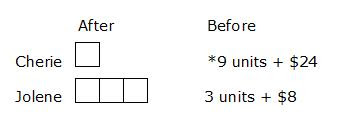Jolene (before) ----- 3 units + \$8
*Cherie (before) had 3 times as much as Jolene -----
(3 x 3 units) + (3 x \$8) = 9 units + \$24

Cherie (before) – Cherie (after) ----- \$40 (spent)
(9 units + \$24) – (1 unit) ----- \$40
8 units + \$24 ------ \$40
8 units ----- \$40 - \$24 = \$16
1 unit ----- \$16 divided by 8 = \$2

Cherie (at first)
9 units + \$24
= (9 x \$2) + \$24
= \$18 + \$24
= \$42

## Saturday, March 21, 2009

### Tao Nan School P5 SA2 2006 Math Q45

A box containing 58 iron balls weighed 2145g . when 46 of the iron balls were removed, the mass of the box and the remaining iron balls weighed 489g. What was the mass of the box?

Solution

Number of iron balls left when 46 were removed
58 – 46 = 12 (iron balls)

Box + 58 iron balls ----- 2145 g
Box + 12 iron balls ----- 489 g

(58 – 12) iron balls ----- 2145 g – 489 g
46 iron balls ----- 1656 g
1 iron ball ----- 1656 g divided by 46 = 36 g

Box + 58 iron balls ----- 2145 g
Box + (58 x 36 g) ----- 2145 g
Box + 2088 g ----- 2145 g
Box ----- 2145 g – 2088 g = 57 g

Answer: The box was 57 g.

## Wednesday, March 18, 2009

### Tao Nan School P5 SA2 2006 Math Q44

ABDE is a parallelogram and BCD is an isosceles triangle. Calculate
a) Angle DBC
b) Angle EAB
The figure below is not drawn to scale.Solution

a) Angle DBC ----- (180 – 42 – 42) degrees = 96 degrees (Answer)

b) Angle EAB = Angle EDB -----
(96 + 42) degrees = 138 degrees (Answer)